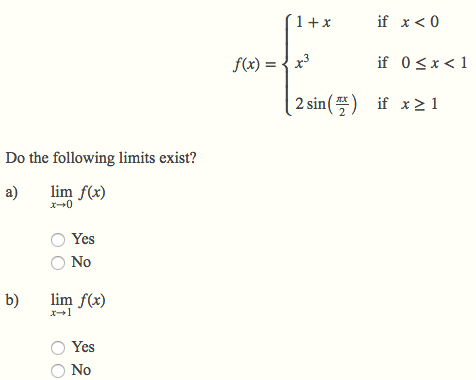D

#### Limit Functions

14 viewed last edited 4 years agoHanna Owens
0http://imgur.com/a/Fowr3 Sorry if you're not allowed to post url/links on the forum but I didnt know how else to format this question. Im not sure how to tackle this question, what exactly is it asking for & how do you go about this question?Mahesh Godavarti
0
Limit at a point exists iff limits coming from the left or right are equal and are equal to the value of the function at that point. I.e. \lim_{x \rightarrow 0} f(x) exists if \lim_{h \rightarrow 0} f(0-h) = f(0) = \lim_{h \rightarrow 0} f(0+h) . Similarly, \lim_{x \rightarrow 1} f(x) exists if \lim_{h \rightarrow 0} f(1-h) = f(1) = \lim_{h \rightarrow 0} f(1 + h) . First, note that f(1 + h) = 2 \sin\left( \frac{\pi (1 + h)}{2} \right), f(1 - h) = (1-h)^3 \text{ and } f(1) = 2 \sin \left( \frac{\pi \cdot 1 }{2} \right) = 2 The \lim_{h \rightarrow 0} f(1+h) = 2, \lim_{h \rightarrow 0} f(1-h) = 1 \text{ and } f(1) = 2 . Therefore, \lim_{x \rightarrow 1} f(x) does not exist. Similarly, \lim_{h \rightarrow 0} f(0 + h) = \lim_{h \rightarrow 0} (0+h)^3 = 0, \lim_{h \rightarrow 0} f(0-h) = \lim_{h \rightarrow 0} 1 - h = 1 \text{ and } f(0) = 0 . Therefore, \lim_{x \rightarrow 0} f(x) does not exist.